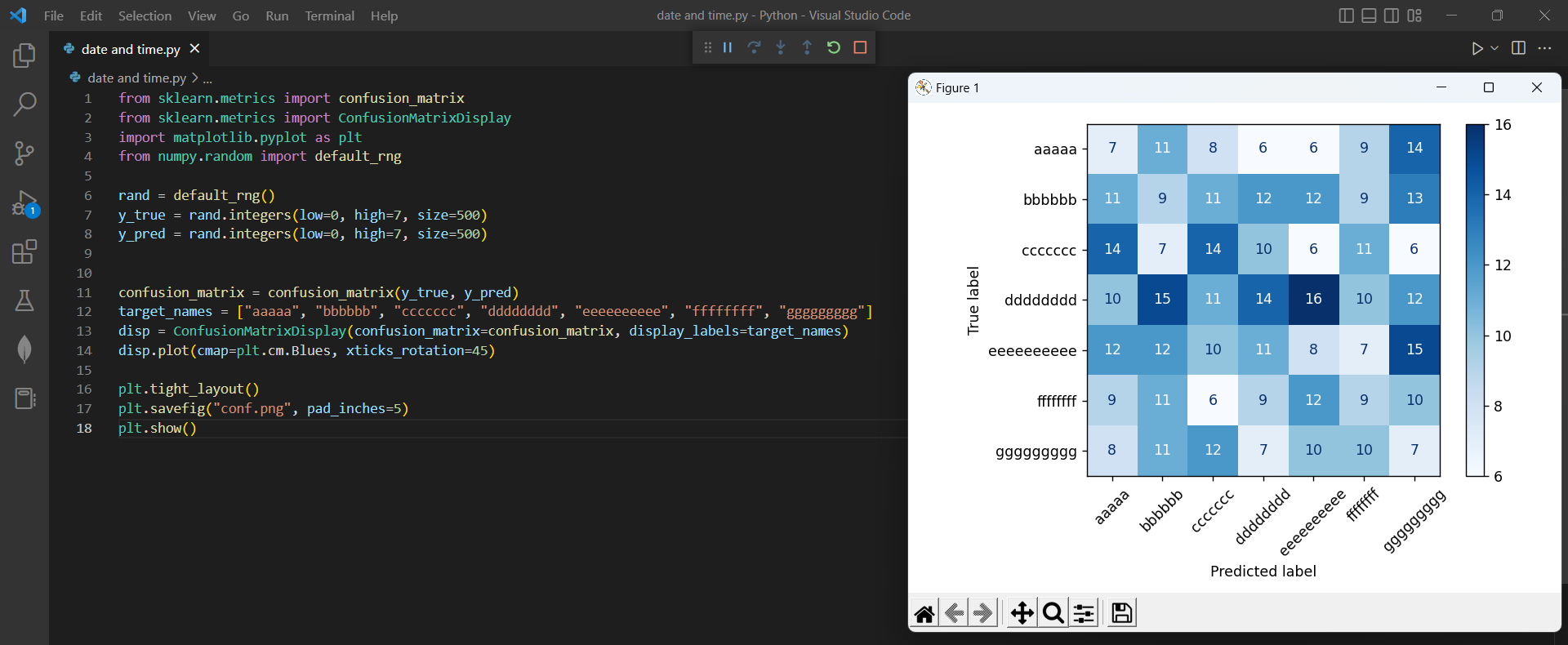# How to solve when labels out of Range in Confusion Matrixby vigneshchennai74Updated: Feb 9, 2023Solution Kit

Most of the time in the confusion matrix the Labels will cut off when we give a long Label name. we came up with a solution for displaying the label without any cut-off, just by using tigh_layout and pad_inches.

A confusion matrix is a performance measurement tool used in machine learning to evaluate the accuracy of a classifier. It is a table that is used to evaluate the correctness of a classifier's predictions by comparing the predicted class labels with the true class labels.The confusion matrix provides a summary of the true positive (TP), false positive (FP), true negative (TN), and false negative (FN) predictions made by a classifier. The entries in the matrix are calculated as follows:

• True Positives (TP): The number of instances where the classifier correctly predicted the positive class.
• False Positives (FP): The number of instances where the classifier incorrectly predicted the positive class, but the true class was negative.
• True Negatives (TN): The number of instances where the classifier correctly predicted the negative class.
• False Negatives (FN): The number of instances where the classifier incorrectly predicted the negative class, but the true class was positive.

The entries in the confusion matrix can be used to calculate various performance metrics, such as accuracy, precision, recall, and F1-score, which give a more comprehensive evaluation of a classifier's performance.

• ConfusionMatrixDisplay: This class provides a convenient way to display the confusion matrix in a graphical format.
• matplotlib.pyplot: This module provides a collection of functions and classes for generating plots and charts using the Matplotlib library.
• default_rng: This function generates a default random number generator that can be used to generate random numbers.

Here is the example of how to solve when the labels are out of the Range:Preview of the output that you will get on running this code from your IDE﻿

### Code

In this solution we have used Scikit-Learn

1. Copy the code using the "Copy" button above, and paste it in a Python file in your IDE.
2. Run the file to get the output

I hope you found this useful. I have added the link to dependent libraries, version information in the following sections.

I found this code snippet by searching for "ConfusionMatrixDisplay (Scikit-Learn) plot labels out of range" in kandi. You can try any such use case!

### Environment Test

I tested this solution in the following versions. Be mindful of changes when working with other versions.

1. The solution is created in Python 3.7.15 version
2. The solution is tested on scikit-learn 1.0.2 version

Using this solution, we are able going to learn if the labels are out of the range while plot we can solve that with the help of rand method in python with simple steps. This process also facilities an easy to use, hassle free method to create a hands-on working version of code which would help of plot label in Python.

### Dependent Library

scikit-learnby scikit-learn

Python54584Version:1.2.2scikit-learn: machine learning in Python

Support
Quality
Security
Reuse

scikit-learnby scikit-learn

Python54584Version:1.2.2License: Permissive (BSD-3-Clause)

scikit-learn: machine learning in Python
Support
Quality
Security